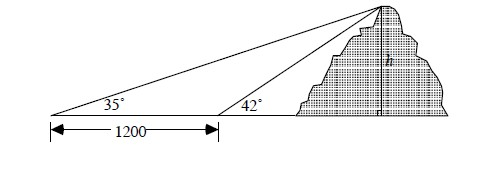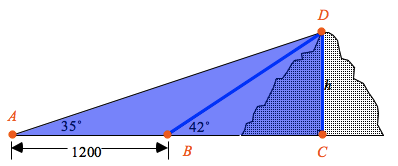### Home > PC > Chapter 3 > Lesson 3.2.1 > Problem3-64

3-64.

To find the height $h$ of Mount St. Melon in the Cantaloupe Mountains, two angle measurements were taken $1200$ feet apart along a direct line toward the mountain. Using these measurements, find the height of the mountain.1. Find angle $ABD$ by subtracting $42$ from $180^\circ$.
2. Now find angle $ADB$. You know the sum of the angles of a triangle $= 180^\circ$.
3. Use the Law of Sines to find length $BD$.
4. Use the $\sin42^\circ$ and $BD$ to find $h$.